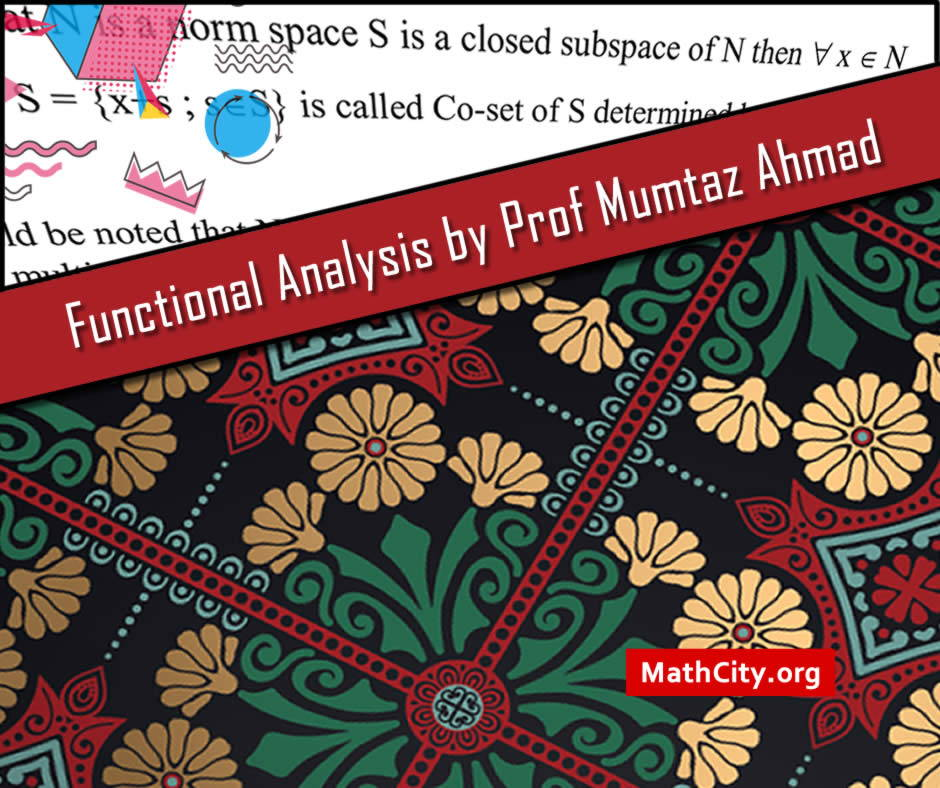# Functional Analysis by Prof Mumtaz AhmadFunctional analysis is a subfield of mathematics that deals with vector space theory and linear algebra. It entails researching the connections between roles, things, incidents, actions, and outcomes. The word “functional” refers to a function whose argument is a function and stems from the calculus of variations. It can be used in many different areas, including approximation theory, calculus of variations, numerical analysis, and differential and integral equations.

These notes contain the important concept of the metric space. A collection of points with a concept of distance between them make up a metric space. A metric or distance function is used to calculate the distance. Numerous elements of mathematical analysis and geometry are studied using metric spaces. The triangle inequality is satisfied by the distance function, which is symmetric and nonnegative. For other concept, there is a need to dig out the notes.

These notes are provided and composed by Mr. Muzammil Tanveer. We are really very thankful to him for providing these notes and appreciates his effort to publish these notes on MathCity.org

#### Summary

• Name: Functional Analysis
• Provider: Mr. Muzammil Tanveer
• Pages: 71 pages
• Format: PDF
• Size: 2.7 MB
• Category: Analysis
• Level: MSc, BS Mathematics
• Metric Space
• Distance between two sets
• Sequence
• Convergent Sequence
• Cauchy Sequence
• Complete Space
• Dense Subset
• Somewhere & Nowhere Dense Subset
• Nested Sequence
• Normed Space
• $l^\infty$-Space
• $l^\infty$ is Banach space
• C-space
• Open ball
• Quotient Space
• Equivalent Norms
• Topological Linear Space
• Linear Operator
• Finite Dimensional Normed Space
• Cauchy Schwarz Inequality
• Orthogonal System
• Generalized form of Pythagorean Theorem
• Dual Space (Conjugate Space)
• Isometric Isomorphism
• Annihilators